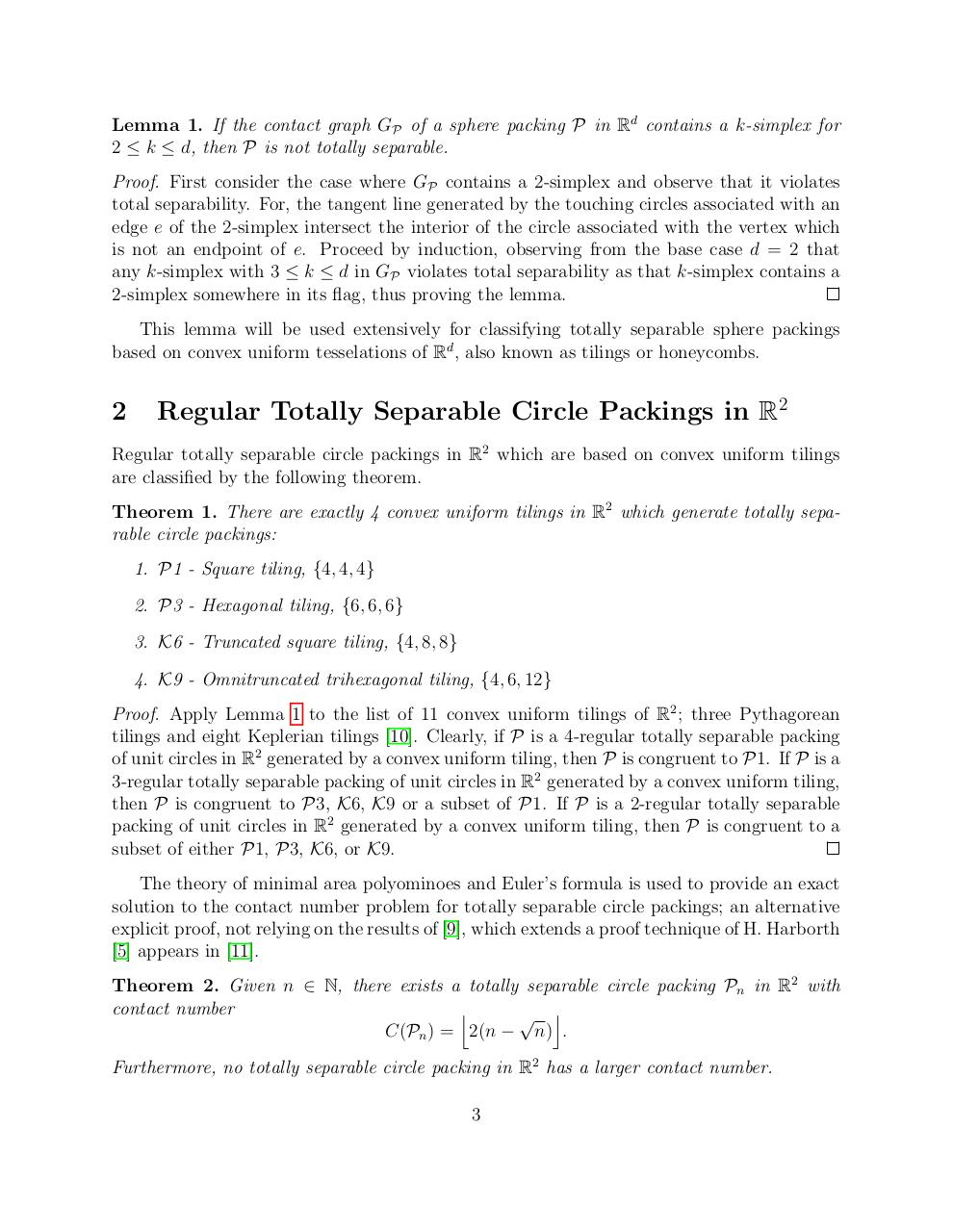# Regular Totally Separable Sphere Packings arXiv.pdfPage 1 2 3 4 5 6 7 8 9 10

#### Text preview

Lemma 1. If the contact graph GP of a sphere packing P in Rd contains a k-simplex for
2 ≤ k ≤ d, then P is not totally separable.
Proof. First consider the case where GP contains a 2-simplex and observe that it violates
total separability. For, the tangent line generated by the touching circles associated with an
edge e of the 2-simplex intersect the interior of the circle associated with the vertex which
is not an endpoint of e. Proceed by induction, observing from the base case d = 2 that
any k-simplex with 3 ≤ k ≤ d in GP violates total separability as that k-simplex contains a
2-simplex somewhere in its flag, thus proving the lemma.
This lemma will be used extensively for classifying totally separable sphere packings
based on convex uniform tesselations of Rd , also known as tilings or honeycombs.

2

Regular Totally Separable Circle Packings in R2

Regular totally separable circle packings in R2 which are based on convex uniform tilings
are classified by the following theorem.
Theorem 1. There are exactly 4 convex uniform tilings in R2 which generate totally separable circle packings:
1. P1 - Square tiling, {4, 4, 4}
2. P3 - Hexagonal tiling, {6, 6, 6}
3. K6 - Truncated square tiling, {4, 8, 8}
4. K9 - Omnitruncated trihexagonal tiling, {4, 6, 12}
Proof. Apply Lemma 1 to the list of 11 convex uniform tilings of R2 ; three Pythagorean
tilings and eight Keplerian tilings . Clearly, if P is a 4-regular totally separable packing
of unit circles in R2 generated by a convex uniform tiling, then P is congruent to P1. If P is a
3-regular totally separable packing of unit circles in R2 generated by a convex uniform tiling,
then P is congruent to P3, K6, K9 or a subset of P1. If P is a 2-regular totally separable
packing of unit circles in R2 generated by a convex uniform tiling, then P is congruent to a
subset of either P1, P3, K6, or K9.
The theory of minimal area polyominoes and Euler’s formula is used to provide an exact
solution to the contact number problem for totally separable circle packings; an alternative
explicit proof, not relying on the results of , which extends a proof technique of H. Harborth
 appears in .
Theorem 2. Given n ∈ N, there exists a totally separable circle packing Pn in R2 with
contact number
j
√ k
C(Pn ) = 2(n − n) .
Furthermore, no totally separable circle packing in R2 has a larger contact number.
3